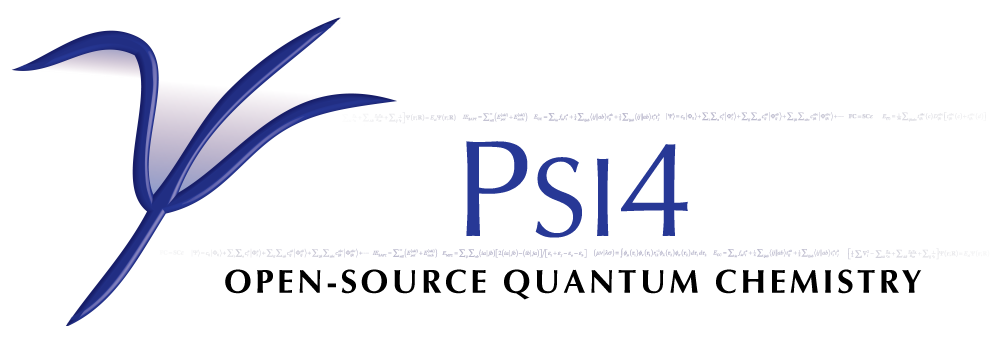# SCF¶

Performs self consistent field (Hartree-Fock and Density Functional Theory) computations. These are the starting points for most computations, so this code is called in most cases.

 `CURRENT ENERGY` `CURRENT REFERENCE ENERGY` `DFT FUNCTIONAL TOTAL ENERGY` `DFT TOTAL ENERGY` `DFT XC ENERGY` `DISPERSION CORRECTION ENERGY` `DOUBLE-HYBRID CORRECTION ENERGY` `EFP IND ENERGY` `EFP TOTAL ENERGY` `HF TOTAL ENERGY` `NUCLEAR REPULSION ENERGY` `ONE-ELECTRON ENERGY` `PCM POLARIZATION ENERGY` `SCF DIPOLE X` `SCF DIPOLE Y` `SCF DIPOLE Z` `SCF ITERATION ENERGY` `SCF QUADRUPOLE XX` `SCF QUADRUPOLE XY` `SCF QUADRUPOLE XZ` `SCF QUADRUPOLE YY` `SCF QUADRUPOLE YZ` `SCF QUADRUPOLE ZZ` `SCF STABILITY EIGENVALUES` (array) `SCF TOTAL ENERGY` `TWO-ELECTRON ENERGY`